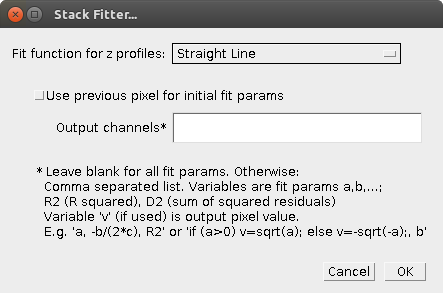# Stack Fitter

This plugin fits the data along the z axis (as obtained with Image>Stacks>Plot z axis profile) for each (x,y) pixel of a stack and creates a new stack with the fit results.

• Supports the built-in ImageJ fit functions (you have modify the code for your own functions).
• Input data are calibrated pixel values (if there is a calibration), also the z axis calibration is taken into account. If there is no calibration for the z axis, note that the z values for the fit are between 0 and NSlices−1, not between 1 and NSlices as the usual numbering of stack slices in ImageJ. This is different from z axis profile plots.
• The output is a stack of fit parameters, functions thereof, and/or data on the quality of the fit for each (x,y) pixel.
• Multi-dimensional stacks (hyperstacks) are not supported.
• Since curve fitting in ImageJ is an iterative process (except for linear regression fits), fitting large amounts of data takes time. Thus, the code puts more emphasis on speed than on retrying the fit with different initial parameters to ensure correct results. This means that in rare cases, the fit quality is worse than when manually doing the same fit with the ImageJ Curve Fitter.

## Dialog Options• Fit function for z profiles
• Use previous pixel for initial fit params
• This should be selected if adjacent pixels (or all pixels) typically have similar z profiles. Then the initial “guess” parameters are taken from the result of the previous pixel.
• When unchecked, ImageJ tries to guess the initial parameter form the data values of each curve.
• This option has no effect for fits using linear regression only (“Straight Line” and everything with “linear regression” in the name).
• Output channels
• Here you can specify the slices of the output stack with a comma-separated list.
• When blank, there is one slice for each fit parameter.
• Otherwise, you can specify which parameter(s) or numbers the slices correspond to:
• Variables ‘‘a, b, c,’’ … are the fit parameters.
• '’R2’’ is the R squared value, the coefficient of determination.
• '’D2’’ is the sum of squared residuals.
• You can also make functions from these; use ‘‘v’’ to specify the function result.
• Example: ‘‘a, -b/(2*c), R2’’’
• Here, the first output stack slice is the ‘a’ parameter,
• the second slice is calculated from the ‘b’ and ‘c’ parameters as ‘‘-b/(2*c)’’, and
• the third slice is R squared.
• Example: ‘‘if (a>0) v=sqrt(a); else v=-sqrt(-a);, b’’
• Here, the first output stack slice is the square root of the ‘a’ parameter, or minus the square root of -a, if a is negative, and
• the second slice gets the fit parameter ‘b’ as pixel values.

## Performance

Fitting a 512×512×10 stack with ‘Exponential with Offset’ needs about a minute on a core i7-6700 (this fit has effectively only one parameter, the two other parameters are calculated directly via linear regression). There is no parallelization except for the parallelization of the ImageJ Minimizer, which uses two threads in parallel to search for minima.

• Source code `Stack_Fitter.java` on GitHub (make sure you download the raw file, use the button near the top right)
• Class file `Stack_Fitter.class` on GitHub

## Installation

• Copy the raw `Stack_Fitter.java` file into the ImageJ plugins folder or a subfolder thereof. Make sure that you name the downloaded file ”Stack_Fitter.java”; uppercase/lowercase matters.
• Compile with “Compile and Run” and press “OK”. Note that “Compile and Run” is currently broken on Fiji; as a workaround use File>New>Script, open the `Stack_Fitter.java` file and press “Run“.
• Alternatively, directly save the .class file `Stack_Fitter.class` into the ImageJ/plugins directory or an immediate subdirectory thereof. Again, make sure that you name the file correctly, uppercase/lowercase matters.
• Use Help>Update Menus or restart ImageJ to make it appear in the Plugins menu (not necessary if you have used the Fiji Script Editor).

## Version History

• 2018-03-23, Michael Schmid: First version